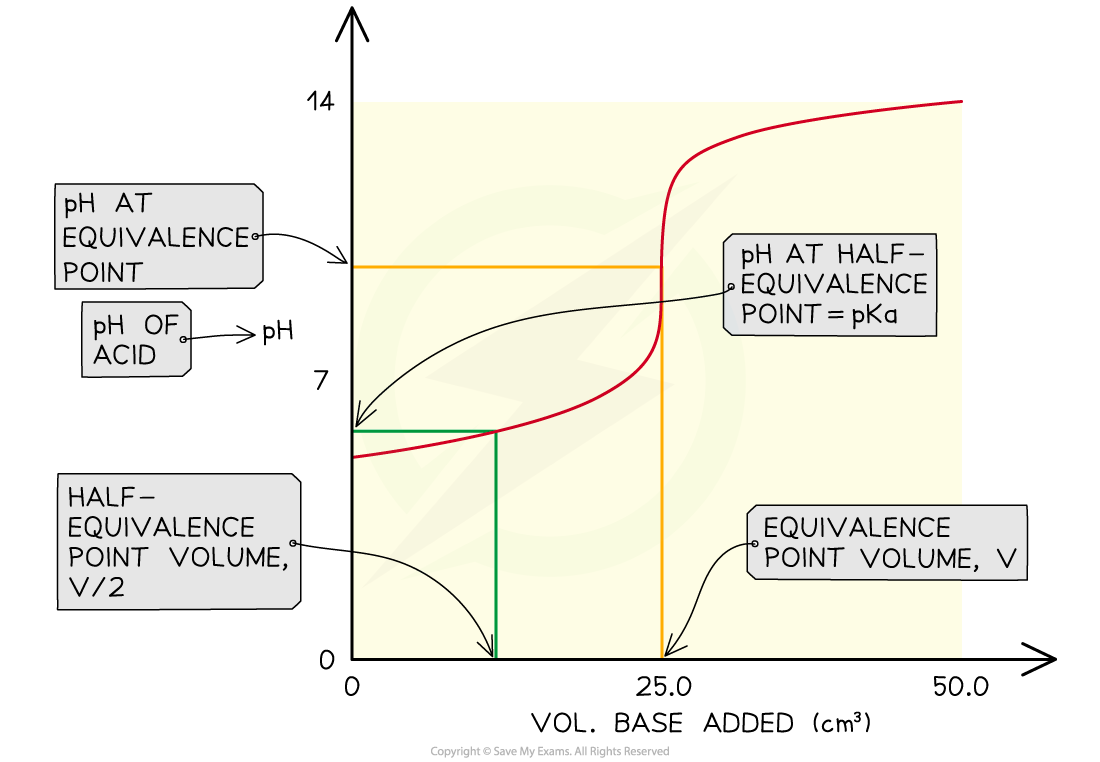# Edexcel A Level Chemistry:复习笔记8.1.1 Finding Ka Values

### Core Practical 9: Finding Ka for a Weak Acid

• The Ka of a weak acid may be determined by finding the pH at the half equivalence point
• At the half equivalence point Ka = [H+]Key steps in the procedure

• A pH probe or meter is calibrated before use
• 25 cm3 of 0.1 mol dm-3 ethanoic acid is pipetted into a conical flask and a few drops of phenolphthalein indicator are added
• A burette is filled with 0.1 mol dm-3 sodium hydroxide solution
• The contents of the conical flask are titrated against the sodium hydroxide until the indicator just turns pink
• A further pipette containing 25 cm3 of the acid is then added to the flask and the pH is measured

Specimen Results

• pH at half equivalence point = 4.75

Analysis

• After the addition of the second portion of acid, the solution is effectively a half-neutralised sample of acid
• This is the half-equivalence point at which the Ka = [H+]
• By measuring the pH at that point you can convert it to [H+] and hence find the Ka of the acid

pH = 4.75

[H+] = 10-pH = 1.8 x 10-5 mol dm-3

∴  Ka = 1.8 x 10-5 mol dm-3

• You can also say that the pKa = pH at the half-equivalence pointThe pH at the half-equivalence point in a weak acid titration gives the pKa of the weak acid

#### Exam Tip

The sources of uncertainty in this experiment include the measurements made by the pipette and burette, and the judgement of the end-point of the titration.Be careful, there is a single uncertainty in the pipette measurements as one reading was taken but there is double the uncertainty for the burette reading as there was the initial and final readings.